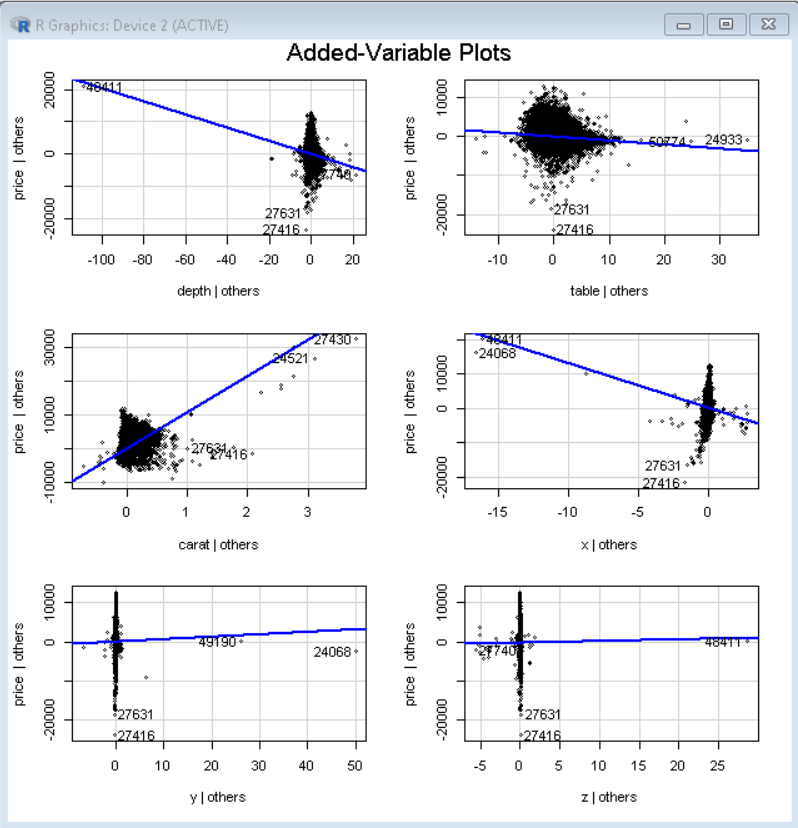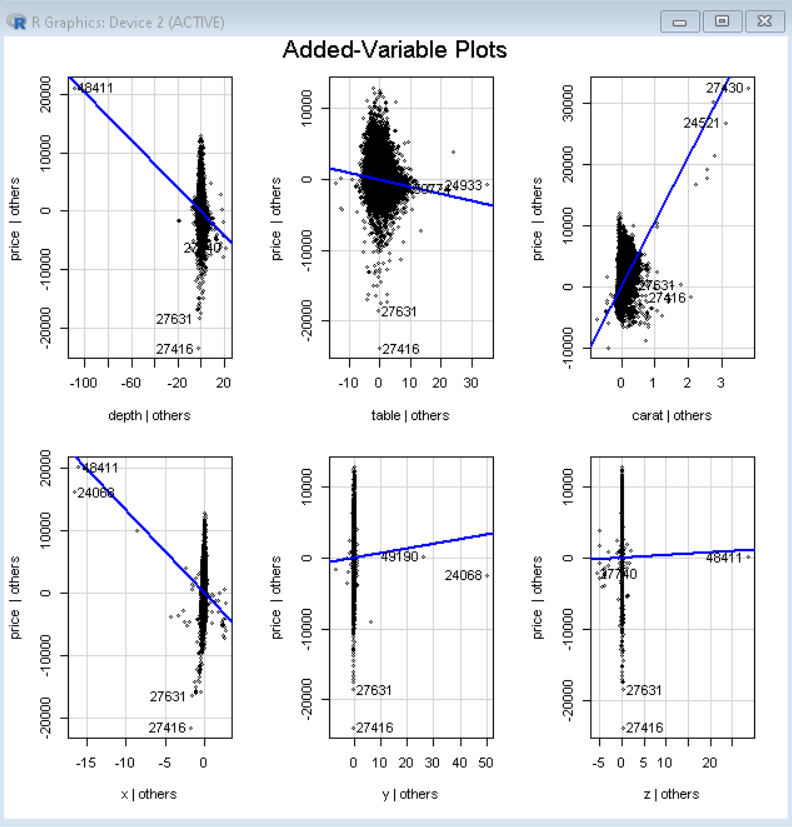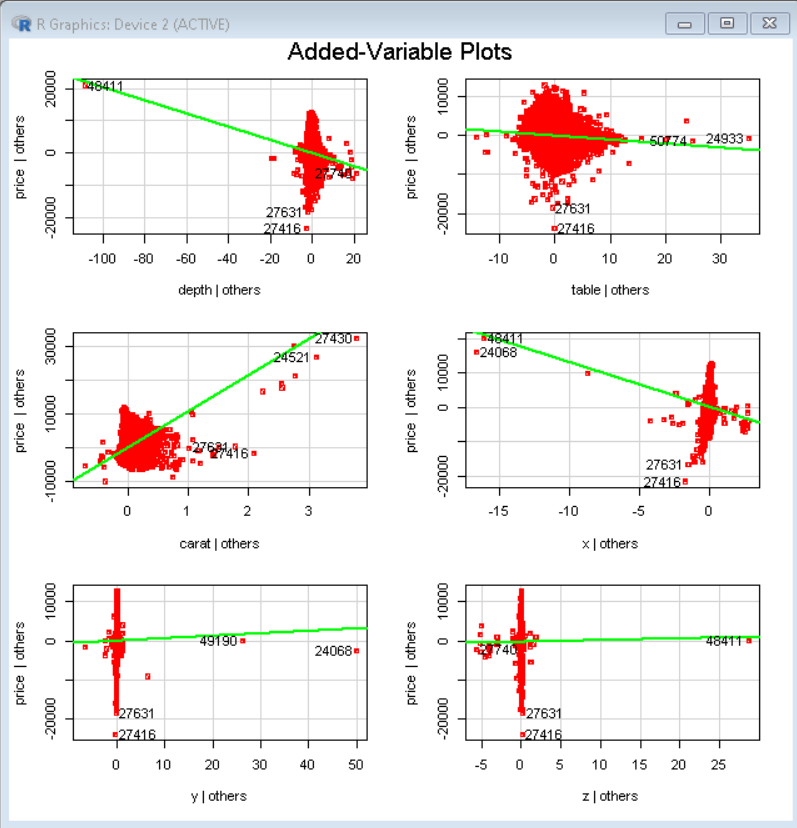Open in App
Not now

# How to Create Added Variable Plots in R?

• Last Updated : 16 Dec, 2021

In this article, we will discuss how to create an added variable plot in the R Programming Language.

The Added variable plot is an individual plot that displays the relationship between a response variable and one predictor variable in a multiple linear regression model while controlling for the presence of other predictor variables in the model. It is also known as the Partial Regression Plot. These Plots allow us to visualize the relationship between each individual predictor variable and the response variable in a model while holding other predictor variables constant.

`Install - install.packages("car")`

Now we select desired cran mirror to install the package and then load the package and use the following syntax to create the Added Variable Plot.

Syntax:

avPlots( linear_model )

where,

• linear_model: determines the model to be visualized.

Example:

Here is a basic added variable plot example made using the avPlots() function. The dataset used in the example is the diamonds dataset which is provided by the R Language natively.

## R

 `# load library car and tidyverse` `library``(car)` `library``(tidyverse)`   `# fit multiple linear regression model ` `# on the data` `linear_model <- ``lm``(price ~ depth + table + carat + ` `                   ``x + y + z, data = diamonds)`   `# visualize linear regression model using ` `# avPlots function` `avPlots``(linear_model)`

Output:## Layout Customization

We can customization the layout of the grid in the avPlots() function by using the layout parameter of the avPlots() function. The layout function takes in a vector as an argument which contains the number of columns and the number of rows variable. These two values determine the layout of the grid.

Syntax:

avPlots( linear_model, layout= c(column, row) )

where,

• linear_model: determines the model to be visualized.
• column: determines the number of columns in the layout grid.
• row: determines the number of rows in the layout grid.

Example:

Here, in this example, we have made added variable plot with a 2X3 grid using layout parameters. The dataset used in the example is the diamonds dataset which is provided by the R Language natively.

## R

 `# load library car and tidyverse` `library``(car)` `library``(tidyverse)`   `# fit multiple linear regression model ` `# on the data` `linear_model <- ``lm``(price ~ depth + table + carat + ` `                   ``x + y + z, data = diamonds)`   `# visualize linear regression model using ` `# avPlots function Use layout parameter for` `# setting the layout of the plot` `avPlots``(linear_model, layout= ``c``(2,3))`

Output:## Color and Shape Customization

We can customize the shape, color, and dimension of the plotted objects i.e. lines and points by using tuning parameters of the avPlots() function. We use col, col.lines, pch, and lwd parameters to change the color of plotted points, the color of plotted lines, the shape of plotted data point, and the width of plotted line respectively.

Syntax:

avPlots( linear_model, col, col.lines, pch, lwd)

where,

• linear_model: determines the model to be visualized.
• col: determines the color of plotted points.
• col.lines: determines the color of plotted lines.
• pch: determines the shape of plotted points.
• lwd: determines the line width for the plotted line.

Example:

Here, is a basic added variable plot with red color points and green color lines with custom shape and width. The dataset used in the example is the diamonds dataset which is provided by the R Language natively.

## R

 `# load library car and tidyverse` `library``(car)` `library``(tidyverse)`   `# fit multiple linear regression model on the data` `linear_model <- ``lm``(price ~ depth + table + carat + ` `                   ``x + y + z, data = diamonds)`   `# visualize linear regression model using avPlots function` `# Use customization parameters to customize the plot` `avPlots``(linear_model, col=``"Red"``, col.lines=``"green"``, pch=14, lwd=2)`

Output:My Personal Notes arrow_drop_up
Related Articles